# Electricity and Magnetism What is magnetism Magnetism is

• Slides: 68Electricity and Magnetism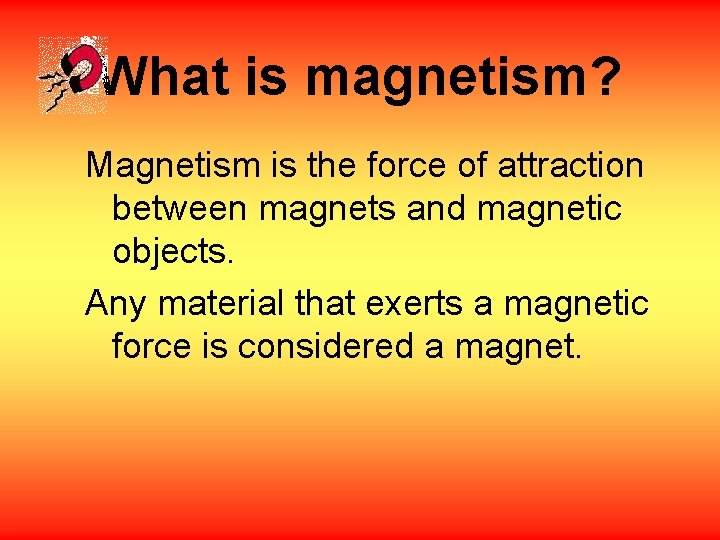What is magnetism? Magnetism is the force of attraction between magnets and magnetic objects. Any material that exerts a magnetic force is considered a magnet.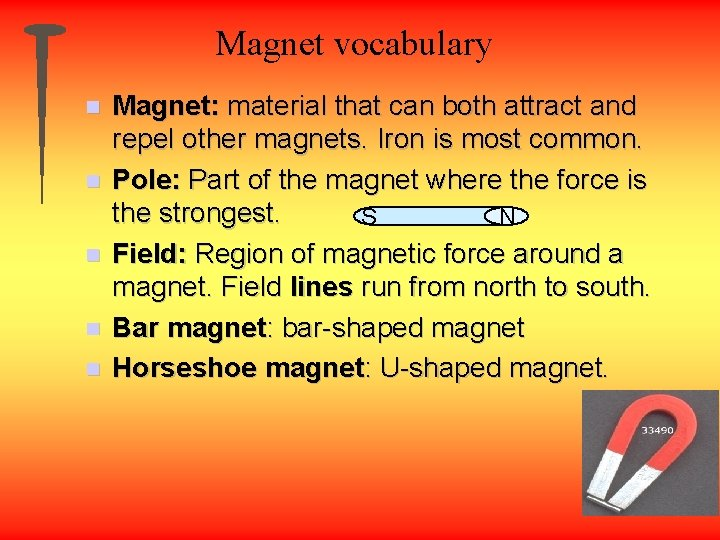Magnet vocabulary n n n Magnet: material that can both attract and repel other magnets. Iron is most common. Pole: Part of the magnet where the force is the strongest. S N Field: Region of magnetic force around a magnet. Field lines run from north to south. Bar magnet: bar-shaped magnet Horseshoe magnet: U-shaped magnet.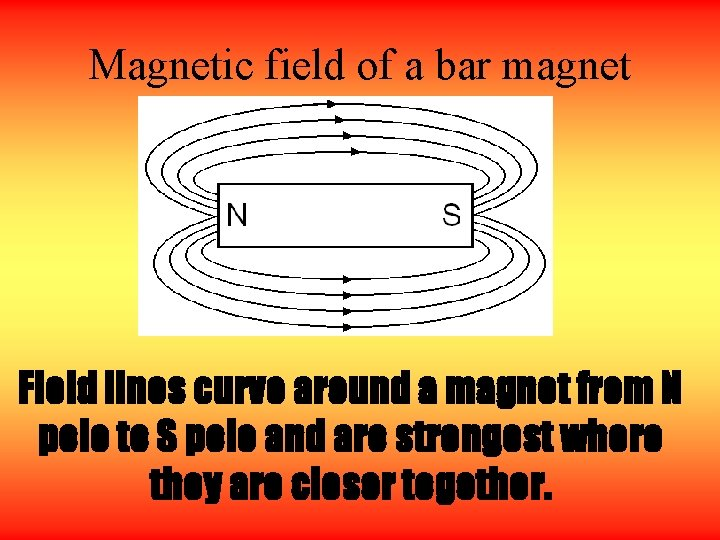Magnetic field of a bar magnet Field lines curve around a magnet from N pole to S pole and are strongest where they are closer together.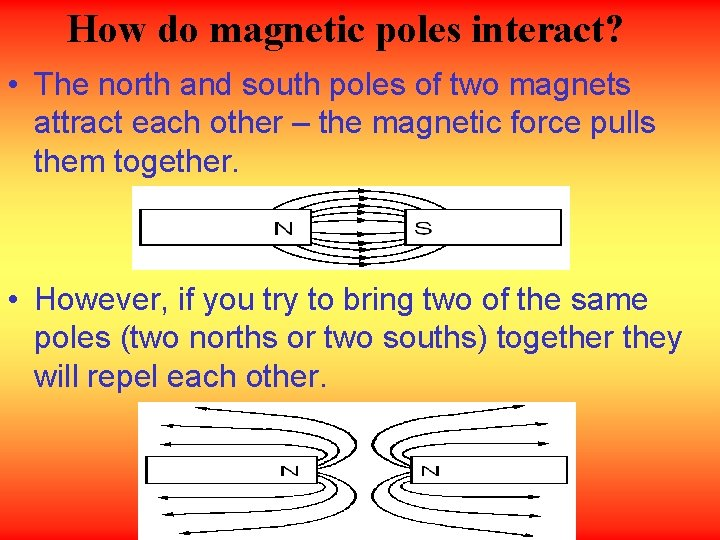How do magnetic poles interact? • The north and south poles of two magnets attract each other – the magnetic force pulls them together. • However, if you try to bring two of the same poles (two norths or two souths) together they will repel each other.What happens if a magnet is broken? When a magnet is broken, two separate magnets are created, each with their own N and S poles!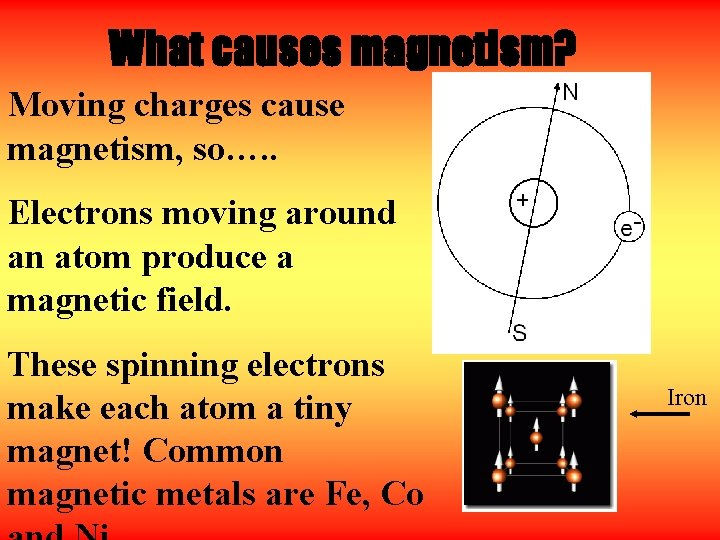What causes magnetism? Moving charges cause magnetism, so…. . Electrons moving around an atom produce a magnetic field. These spinning electrons make each atom a tiny magnet! Common magnetic metals are Fe, Co IronWhat are magnetic domains? • In most materials the magnetic fields of atoms point in random directions. • A cluster of billions of atoms that all have magnetic fields lined up in the same way is known as a magnetic domain.How is magnetism related to domains? In a magnetized material, all or most of the domains are arranged in the same direction –the magnetic properties of a substance depends on the electron arrangement in the atoms.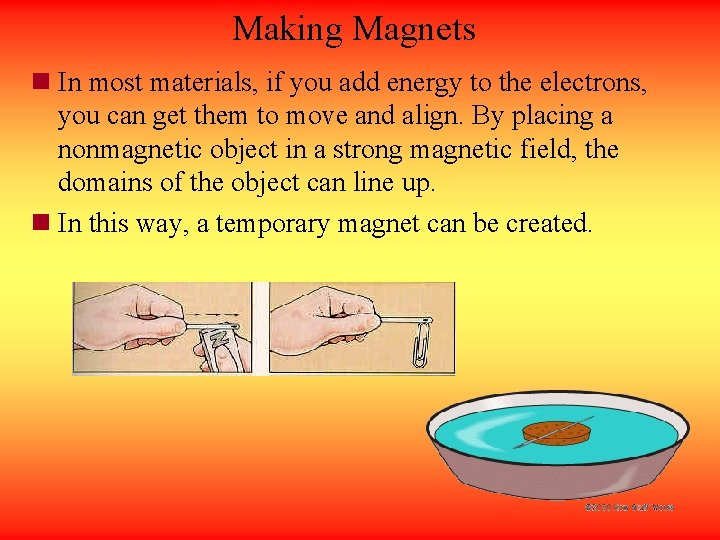Making Magnets n In most materials, if you add energy to the electrons, you can get them to move and align. By placing a nonmagnetic object in a strong magnetic field, the domains of the object can line up. n In this way, a temporary magnet can be created.Can a magnet be destroyed? • Heating a magnet or striking a magnet can cause the domains to become misaligned and a magnet can lose its magnetism. • Above a certain temperature, magnets will lose their magnetism.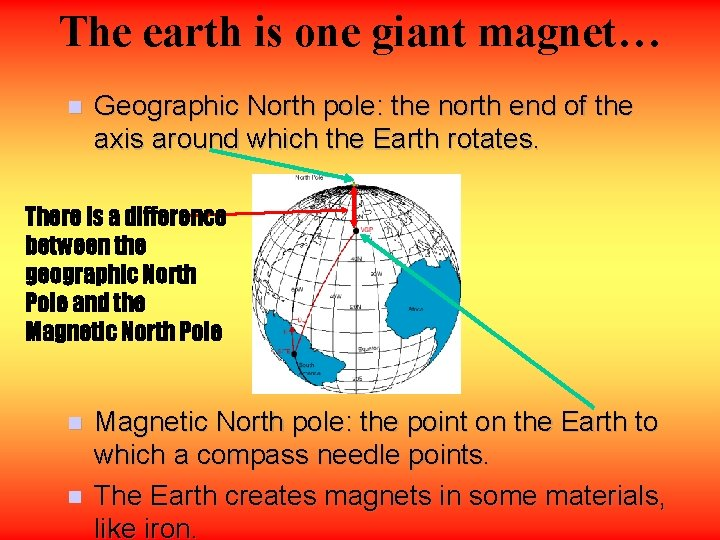The earth is one giant magnet… n Geographic North pole: the north end of the axis around which the Earth rotates. There is a difference between the geographic North Pole and the Magnetic North Pole n n Magnetic North pole: the point on the Earth to which a compass needle points. The Earth creates magnets in some materials, like iron.The earth is surrounded by a magnetic field The earth’s magnetic field affects the movement of electrically charged particles in space.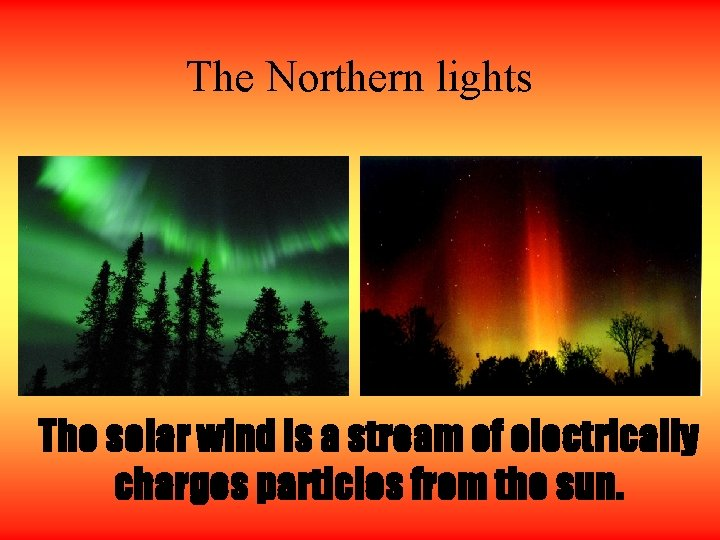The Northern lights The solar wind is a stream of electrically charges particles from the sun.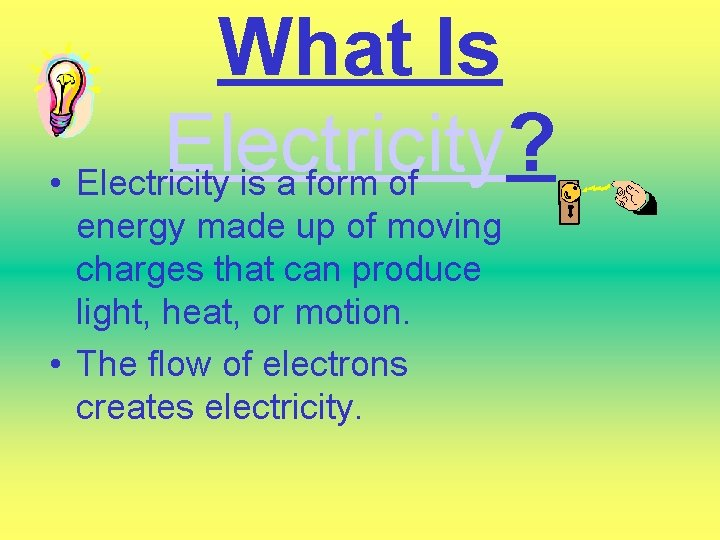What Is Electricity? • Electricity is a form of energy made up of moving charges that can produce light, heat, or motion. • The flow of electrons creates electricity.Kinds of Electricity Static • An electric charge that collects or builds up on the surface of an object, but does not flow. • Examples: – Shocking – Lightning – Plastic wrap Current • The flow of electric charges carried through a material such as wire or other conductors. • Examples: – Power lines – Computers – AppliancesHow does static electricity build up? • A neutral object can become charged by gaining or losing electrons. • An object that loses electrons will become positively charged, whereas an object that gains electrons becomes negatively charged.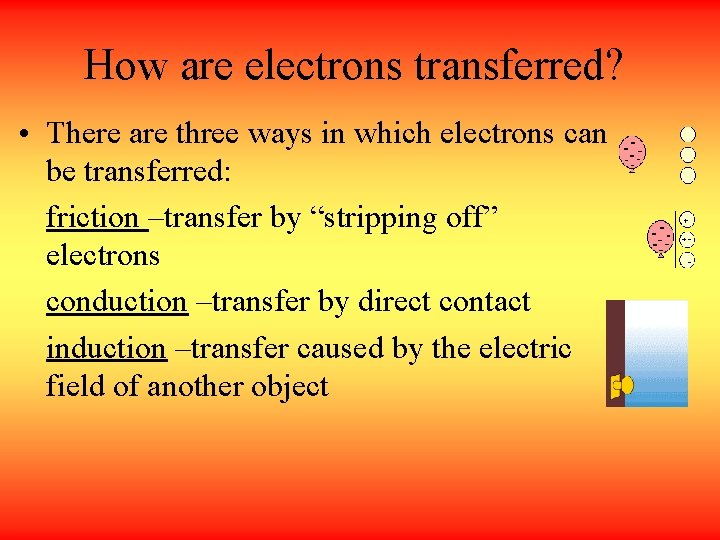How are electrons transferred? • There are three ways in which electrons can be transferred: friction –transfer by “stripping off” electrons conduction –transfer by direct contact induction –transfer caused by the electric field of another object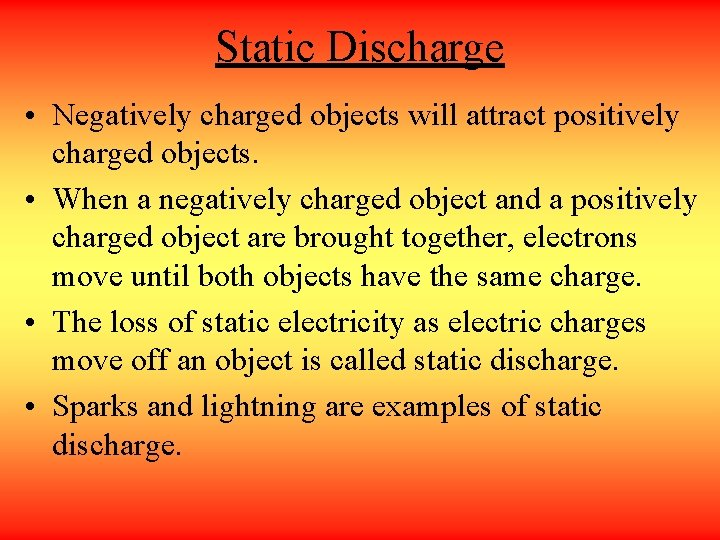Static Discharge • Negatively charged objects will attract positively charged objects. • When a negatively charged object and a positively charged object are brought together, electrons move until both objects have the same charge. • The loss of static electricity as electric charges move off an object is called static discharge. • Sparks and lightning are examples of static discharge.Electrical Fields Charges are similar to magnets -they exert a force at a distance in the area around the charged object creating an electrical field. Electrical fields pull or push on any other charges in their vicinity.Electrical Fields Electrical fields are represented by arrows pointing in the direction a positive charge would be forced to move.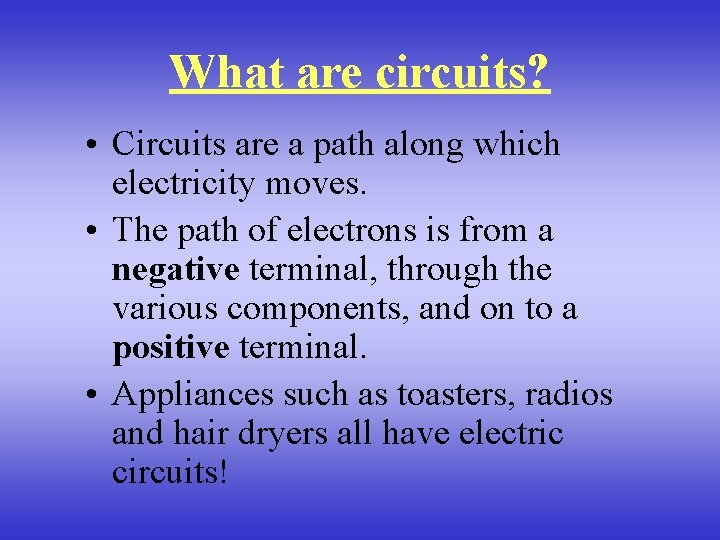What are circuits? • Circuits are a path along which electricity moves. • The path of electrons is from a negative terminal, through the various components, and on to a positive terminal. • Appliances such as toasters, radios and hair dryers all have electric circuits!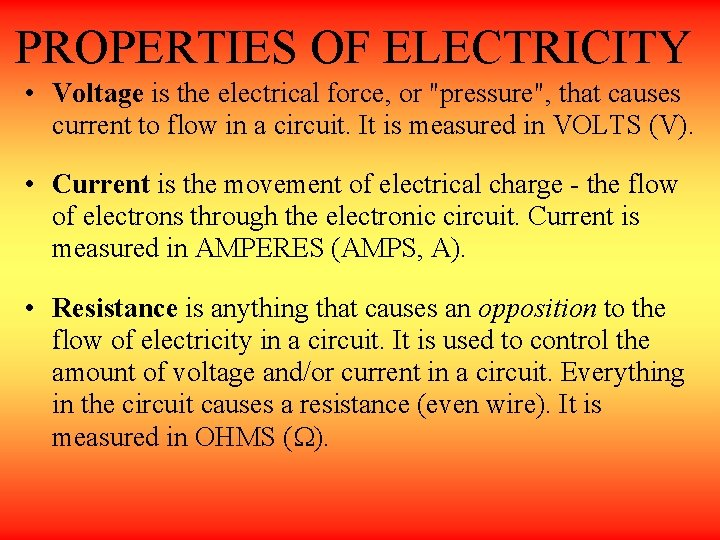PROPERTIES OF ELECTRICITY • Voltage is the electrical force, or "pressure", that causes current to flow in a circuit. It is measured in VOLTS (V). • Current is the movement of electrical charge - the flow of electrons through the electronic circuit. Current is measured in AMPERES (AMPS, A). • Resistance is anything that causes an opposition to the flow of electricity in a circuit. It is used to control the amount of voltage and/or current in a circuit. Everything in the circuit causes a resistance (even wire). It is measured in OHMS (W).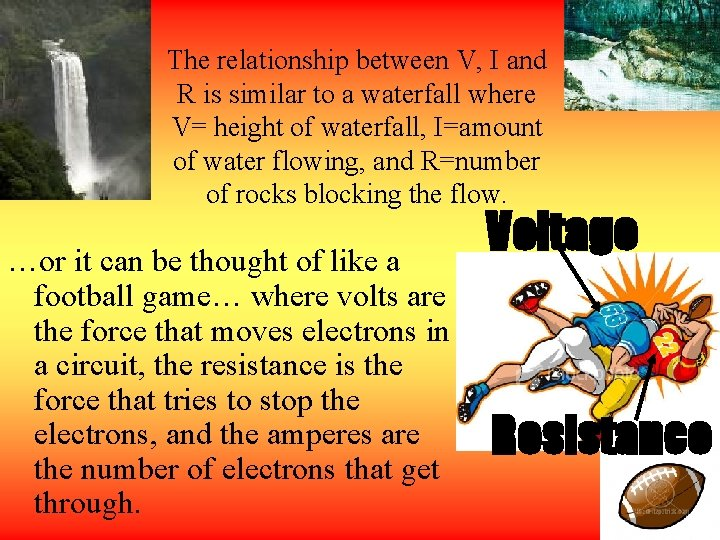The relationship between V, I and R is similar to a waterfall where V= height of waterfall, I=amount of water flowing, and R=number of rocks blocking the flow. …or it can be thought of like a football game… where volts are the force that moves electrons in a circuit, the resistance is the force that tries to stop the electrons, and the amperes are the number of electrons that get through. Voltage ResistanceV I R What is the relationship between these properties? • The relationship between these properties is given by “Ohm’s Law”: V=IR where V=battery voltage, I=current and R=resistance • Resistance is measured in Ohms (W) Current is measured in Amperes (A) • Example 1: What is I for a circuit with a 12 V battery and R = 2 W? I = V/R = 6 A • Example 2: What is I for a circuit where resistance is 2 W and the battery has 6 V? Therefore, I = V/R = 3 AKinds of Circuits Open/Closed Open circuits do not have a complete path to follow. The power cannot pass from the source to the receiver. Closed circuits allow electricity to pass. *Light bulb when it is turned off (open). *Light bulb when it is turned on (closed). Series Parallel These circuits These have a single circuits have complete path more than for electricity one path for to follow. electricity to flow through. *Christmas tree lights *Your house *Power linesCircuit Materials • Conductor: a conductor is a material (usually a metal such as copper) that allows electrical current to pass easily through. (ex: copper) • Insulator: a material that is a poor conductor and has electrons that are tightly bound to individual atoms. (ex: neon) • Semiconductor: a material having electrical properties somewhere between those of good electrical conductors and insulators. (ex: silicon)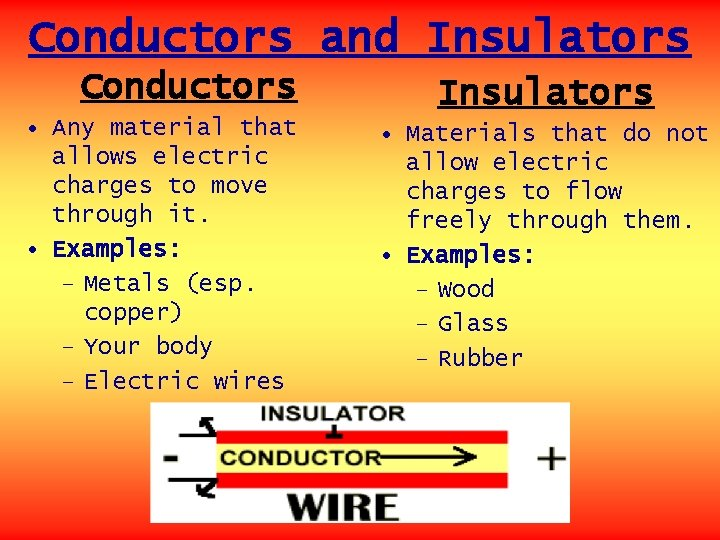Conductors and Insulators Conductors • Any material that allows electric charges to move through it. • Examples: – Metals (esp. copper) – Your body – Electric wires Insulators • Materials that do not allow electric charges to flow freely through them. • Examples: – Wood – Glass – Rubber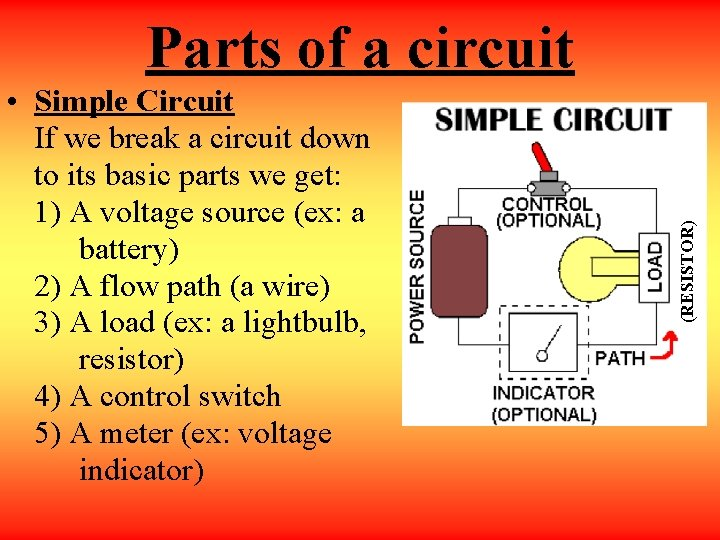• Simple Circuit If we break a circuit down to its basic parts we get: 1) A voltage source (ex: a battery) 2) A flow path (a wire) 3) A load (ex: a lightbulb, resistor) 4) A control switch 5) A meter (ex: voltage indicator) (RESISTOR) Parts of a circuit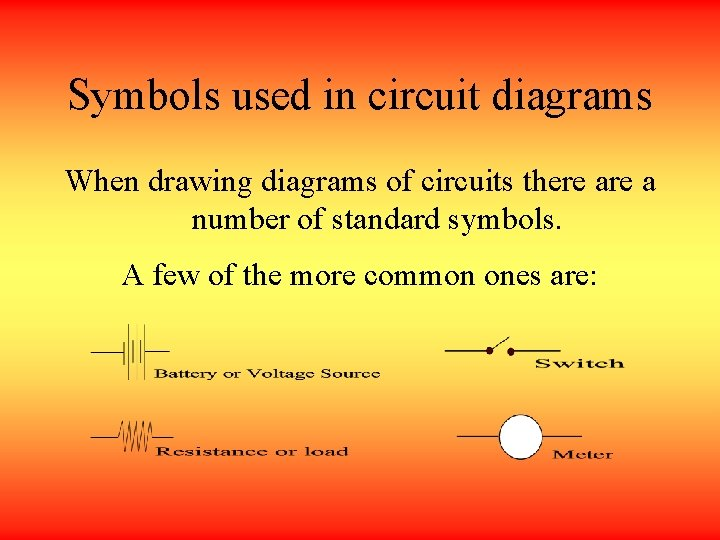Symbols used in circuit diagrams When drawing diagrams of circuits there a number of standard symbols. A few of the more common ones are: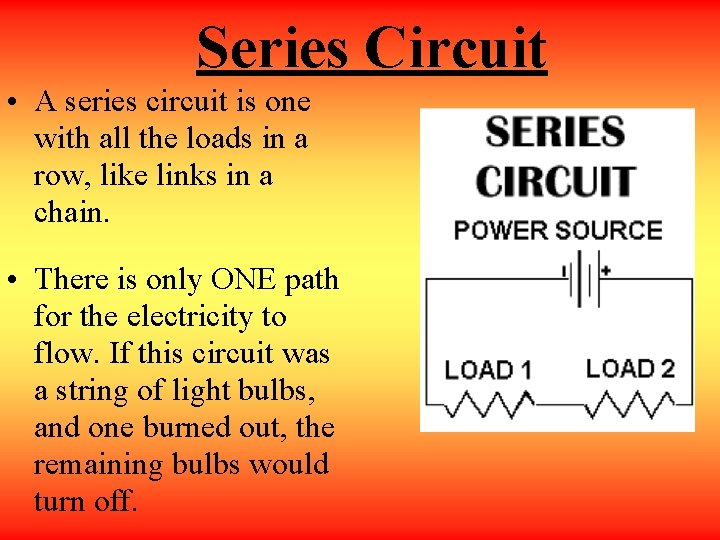Series Circuit • A series circuit is one with all the loads in a row, like links in a chain. • There is only ONE path for the electricity to flow. If this circuit was a string of light bulbs, and one burned out, the remaining bulbs would turn off.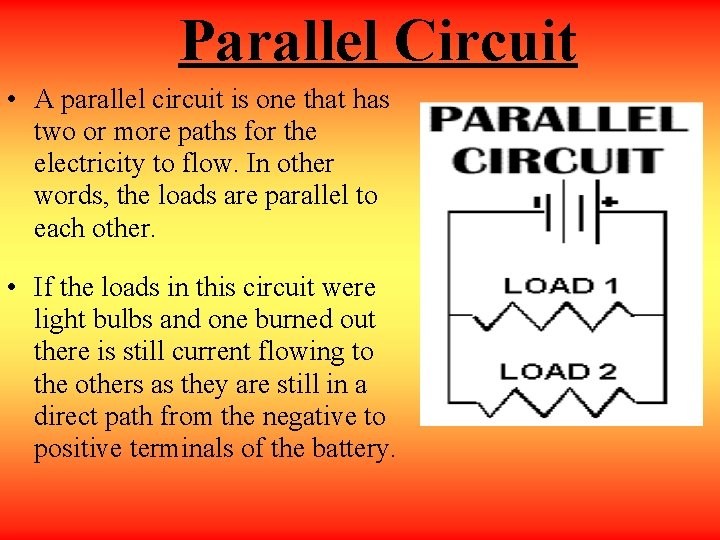Parallel Circuit • A parallel circuit is one that has two or more paths for the electricity to flow. In other words, the loads are parallel to each other. • If the loads in this circuit were light bulbs and one burned out there is still current flowing to the others as they are still in a direct path from the negative to positive terminals of the battery.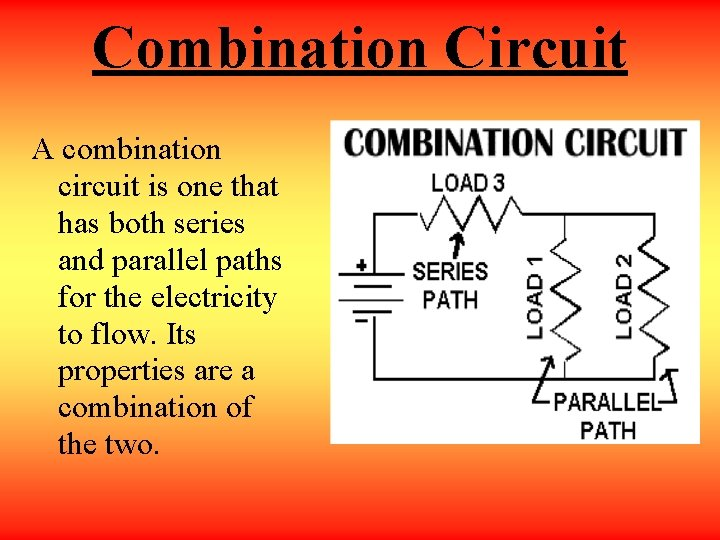Combination Circuit A combination circuit is one that has both series and parallel paths for the electricity to flow. Its properties are a combination of the two.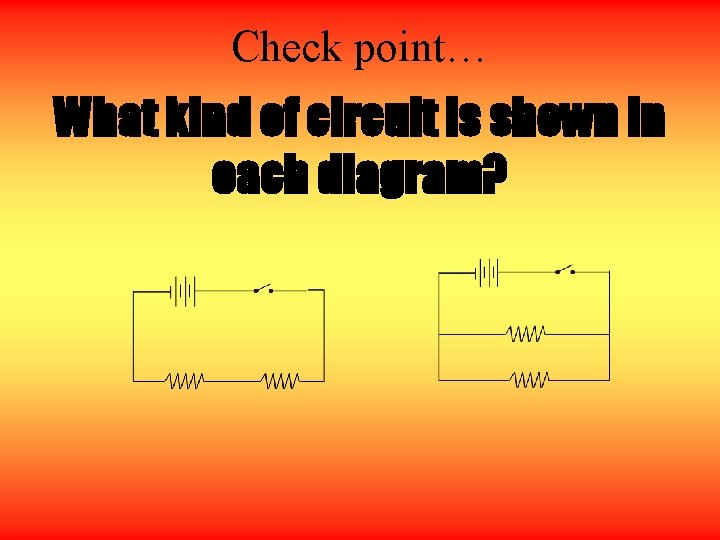Check point… What kind of circuit is shown in each diagram?Practice Problem • The brightness of the bulbs in a circuit indicates the amount of current flowing through the bulbs. • How will the brightness of the bulbs change as we add more in series? + + + • What does this imply about the current in the circuit? Lights become dimmer as bulbs are added because there is more total resistance. This means that the current is reduced.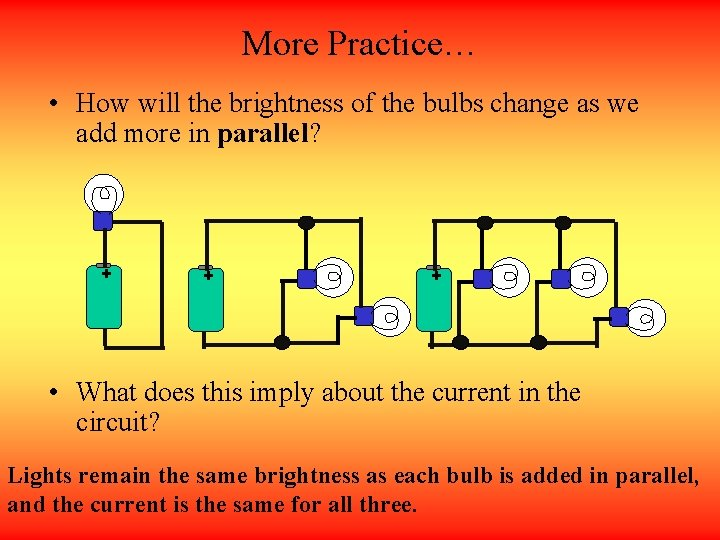More Practice… • How will the brightness of the bulbs change as we add more in parallel? + + + • What does this imply about the current in the circuit? Lights remain the same brightness as each bulb is added in parallel, and the current is the same for all three.Key Concepts • Bulbs in series represent a source of resistance to current flow, similar to boulders in a stream which can block the flow of water. Adding bulbs will SLOW the FLOW and thus reduce current. • Bulbs in parallel offer additional pathways for current flow, similar to branches of a stream which can carry more water. Adding bulbs will not decrease flow, so current is the same for all paths.More practice… A • Is this circuit series or parallel? • Combination circuit • How do the bulbs compare in brightness? • A is brighter than B and C • How will the brightness of A and B change if bulb C is removed? • A will stay the same, but B will become brighter. • How will B and C change if A is removed? • They will become brighter B + C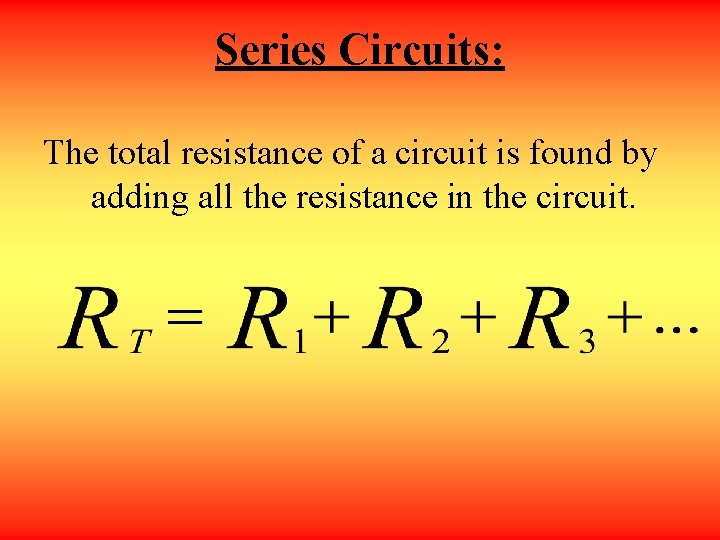Series Circuits: The total resistance of a circuit is found by adding all the resistance in the circuit.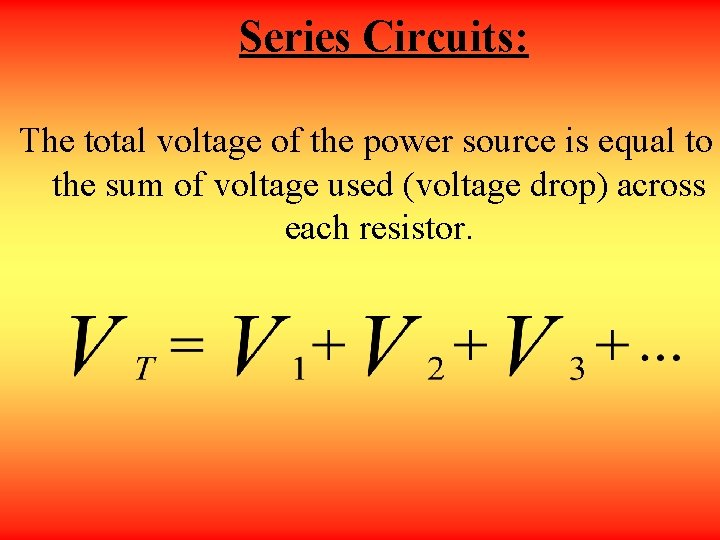Series Circuits: The total voltage of the power source is equal to the sum of voltage used (voltage drop) across each resistor.Series Circuits: The total current through the circuit is equal to the current across each resistor. IT = I 1 = I 2 = I 3 = …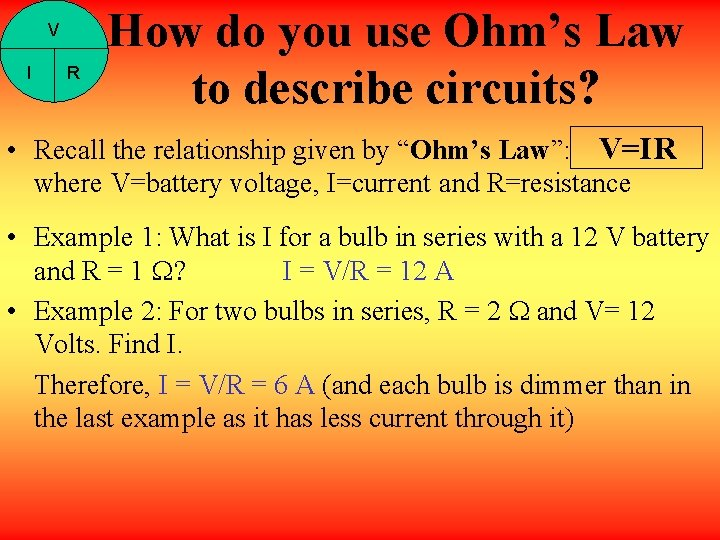V I R How do you use Ohm’s Law to describe circuits? • Recall the relationship given by “Ohm’s Law”: V=IR where V=battery voltage, I=current and R=resistance • Example 1: What is I for a bulb in series with a 12 V battery and R = 1 W? I = V/R = 12 A • Example 2: For two bulbs in series, R = 2 W and V= 12 Volts. Find I. Therefore, I = V/R = 6 A (and each bulb is dimmer than in the last example as it has less current through it)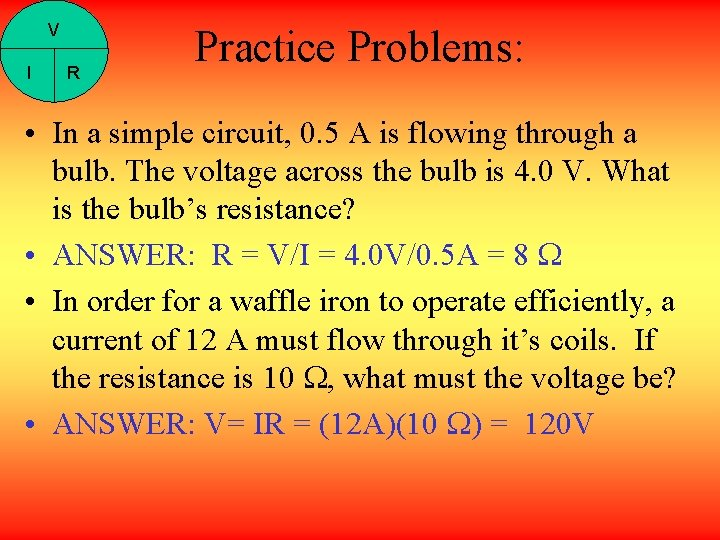V I R Practice Problems: • In a simple circuit, 0. 5 A is flowing through a bulb. The voltage across the bulb is 4. 0 V. What is the bulb’s resistance? • ANSWER: R = V/I = 4. 0 V/0. 5 A = 8 W • In order for a waffle iron to operate efficiently, a current of 12 A must flow through it’s coils. If the resistance is 10 W, what must the voltage be? • ANSWER: V= IR = (12 A)(10 W) = 120 V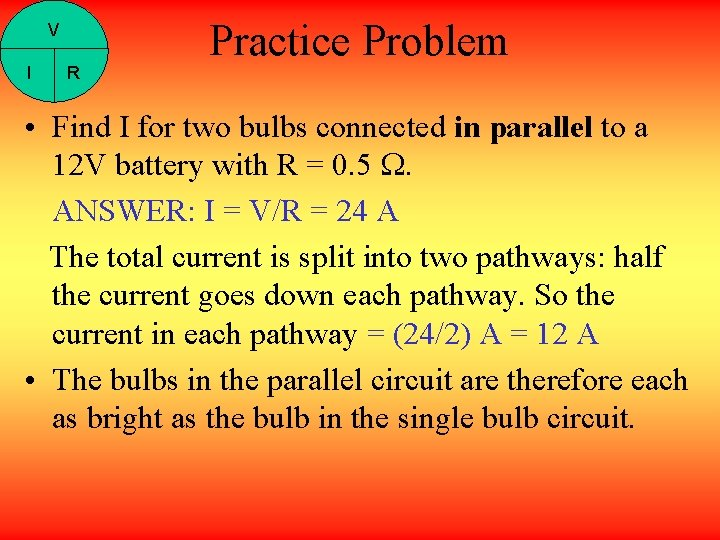V I R Practice Problem • Find I for two bulbs connected in parallel to a 12 V battery with R = 0. 5 W. ANSWER: I = V/R = 24 A The total current is split into two pathways: half the current goes down each pathway. So the current in each pathway = (24/2) A = 12 A • The bulbs in the parallel circuit are therefore each as bright as the bulb in the single bulb circuit.Key Concepts • A battery is a source of constant voltage, for example 1. 5 volts or 12 volts (labeled on the battery). • The current (I) flowing from the battery depends on its voltage (V) and the total resistance (R) in the circuit. • More bulbs in series = more resistance • More parallel pathways = less resistance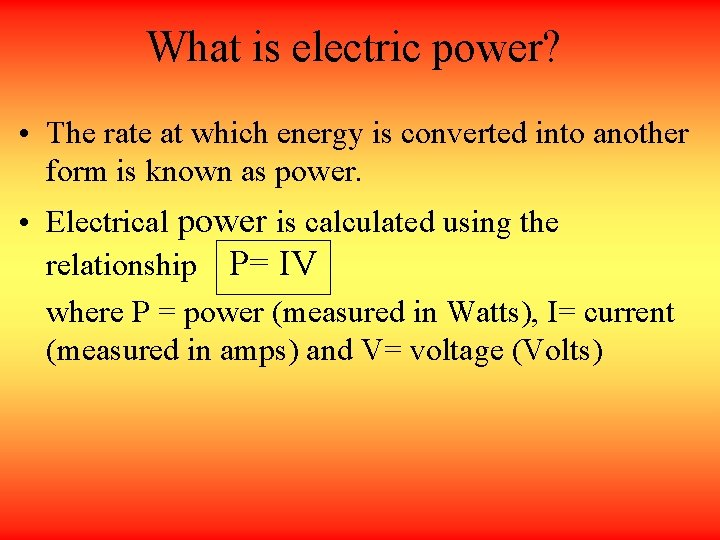What is electric power? • The rate at which energy is converted into another form is known as power. • Electrical power is calculated using the relationship P= IV where P = power (measured in Watts), I= current (measured in amps) and V= voltage (Volts)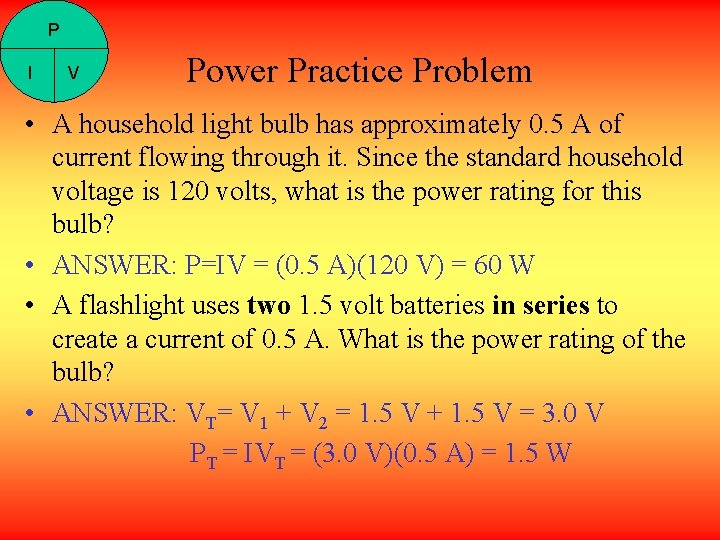P I V Power Practice Problem • A household light bulb has approximately 0. 5 A of current flowing through it. Since the standard household voltage is 120 volts, what is the power rating for this bulb? • ANSWER: P=IV = (0. 5 A)(120 V) = 60 W • A flashlight uses two 1. 5 volt batteries in series to create a current of 0. 5 A. What is the power rating of the bulb? • ANSWER: VT= V 1 + V 2 = 1. 5 V + 1. 5 V = 3. 0 V PT = IVT = (3. 0 V)(0. 5 A) = 1. 5 WSummary of Terms and Units (voltage)Direct Current • A current is considered to be one-directional if it always maintains the same direction of flow; it is two-directional if it changes direction. • An example of a DC current source is a dry cell battery. • http: //videos. howstuffworks. com/hsw/19268 chemistry-connections-primary-and-secondarycells-video. htm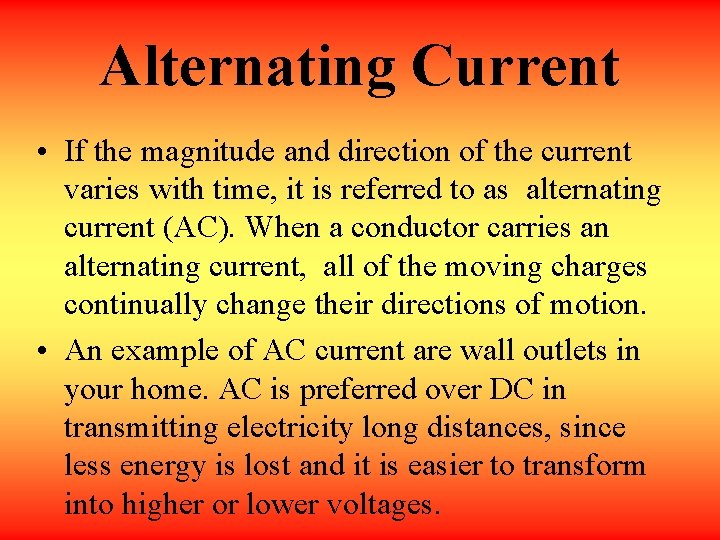Alternating Current • If the magnitude and direction of the current varies with time, it is referred to as alternating current (AC). When a conductor carries an alternating current, all of the moving charges continually change their directions of motion. • An example of AC current are wall outlets in your home. AC is preferred over DC in transmitting electricity long distances, since less energy is lost and it is easier to transform into higher or lower voltages.Magnets and electricity • Magnets are used to generate, or produce, electricity. • Spinning a coil of wire inside a magnetic field produces an electric force between the ends of the coil. In other words, a moving magnet can produce electricity. • In a similar way, an electric current produces a magnetic field around it. In other words, a moving charge forms a magnet and creates a magnetic field.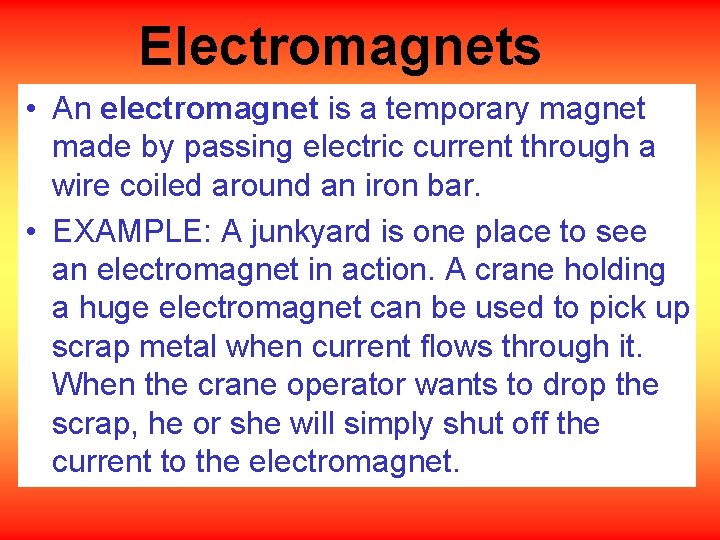Electromagnets • An electromagnet is a temporary magnet made by passing electric current through a wire coiled around an iron bar. • EXAMPLE: A junkyard is one place to see an electromagnet in action. A crane holding a huge electromagnet can be used to pick up scrap metal when current flows through it. When the crane operator wants to drop the scrap, he or she will simply shut off the current to the electromagnet.Strength of Electromagnets Forming a wire into a loop reinforces the field in the center of the loop, repeated loops increase the strength of the magnetic field. Adding an Iron core to the loops further strengthens the magnetic field due to the alignment of the domains in the iron.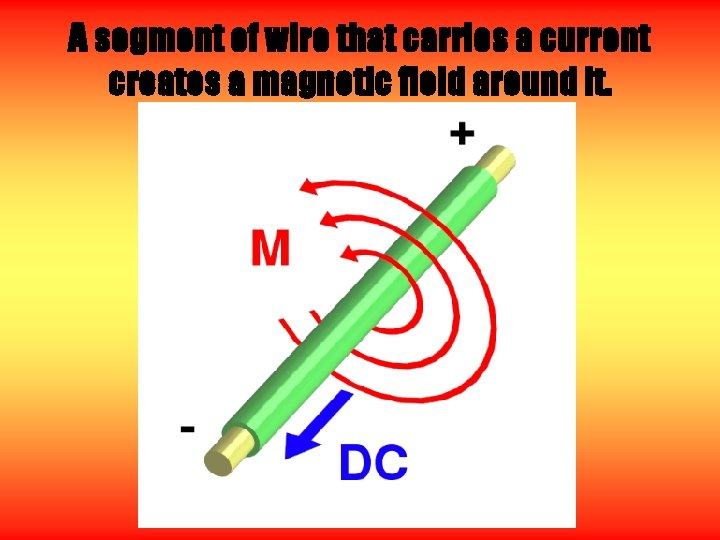A segment of wire that carries a current creates a magnetic field around it.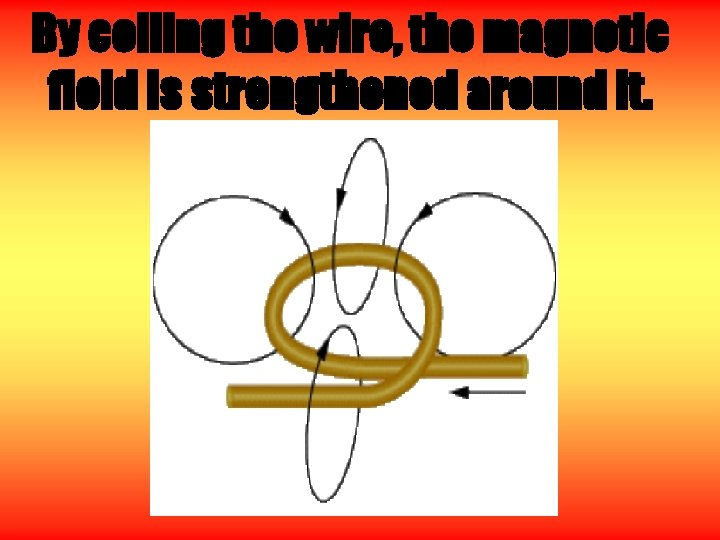By coiling the wire, the magnetic field is strengthened around it.Magnetic field lines around an electromagnet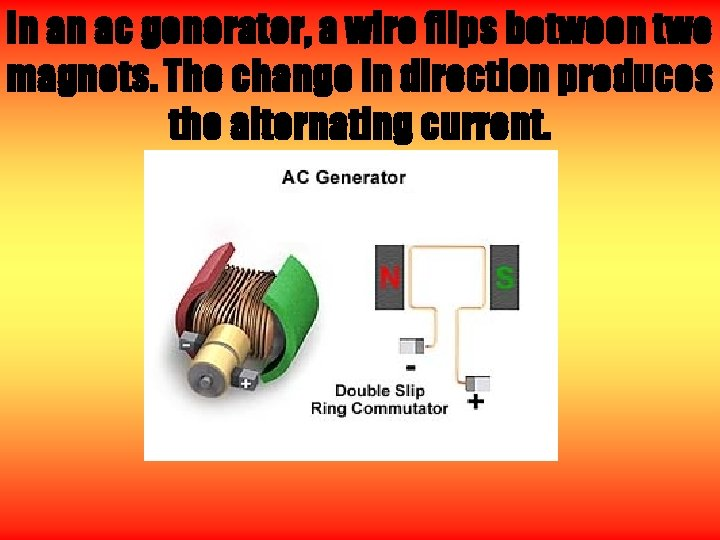In an ac generator, a wire flips between two magnets. The change in direction produces the alternating current.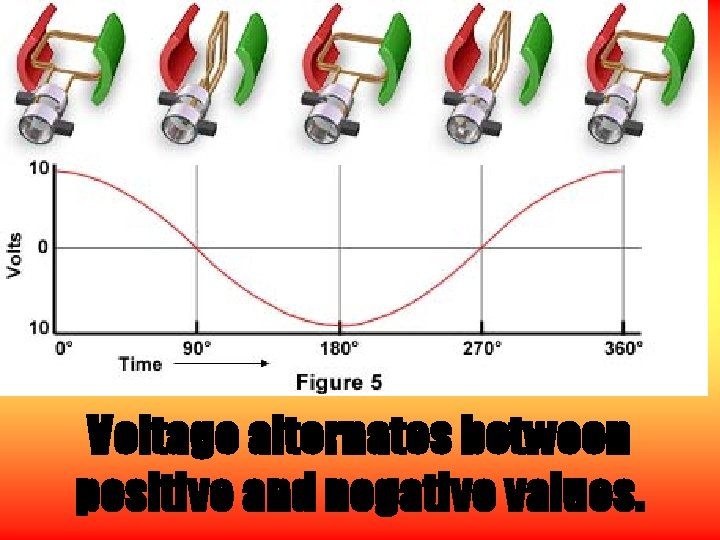Voltage alternates between positive and negative values.THE END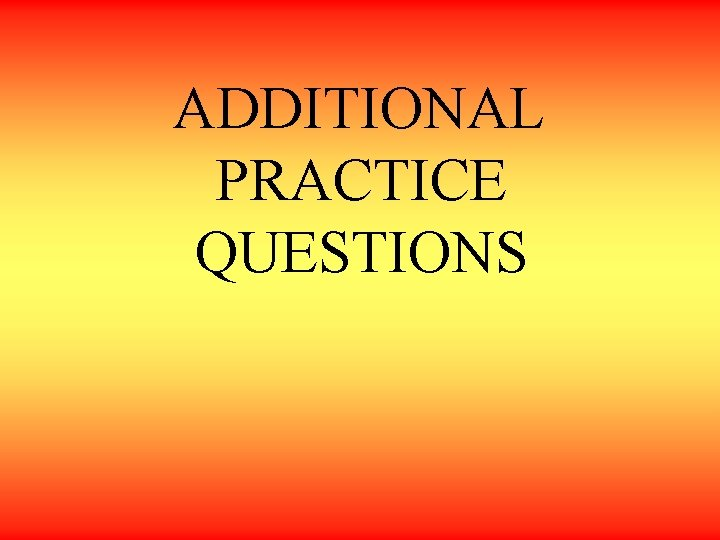NAME THE METAL THAT IS THE BEST CONDUCTOR OF ELECTRICITY: NAME THREE GOOD INSULATING MATERIALS: WHAT ARE SOME GOOD INSULATORS? NAME THREE ESSENTIAL PARTS OF A SIMPLE ELECTRICAL CIRCUIT: IN A SERIES CIRCUIT THERE ARE HOW MANY PATHS FOR CURRENT FLOW?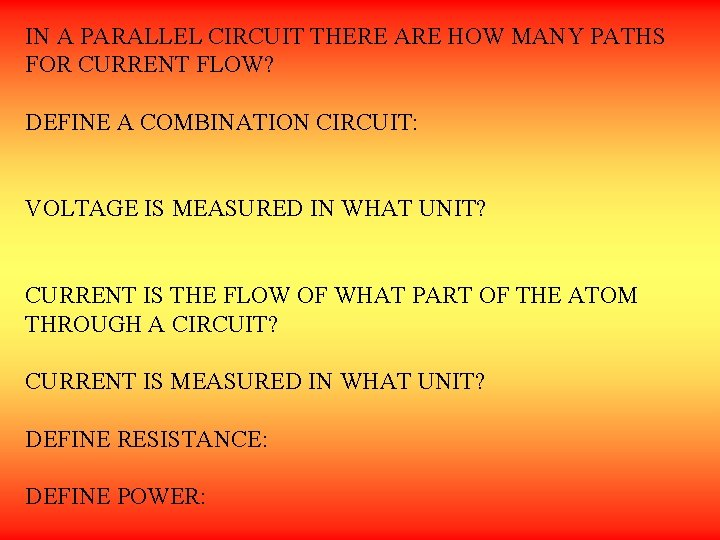IN A PARALLEL CIRCUIT THERE ARE HOW MANY PATHS FOR CURRENT FLOW? DEFINE A COMBINATION CIRCUIT: VOLTAGE IS MEASURED IN WHAT UNIT? CURRENT IS THE FLOW OF WHAT PART OF THE ATOM THROUGH A CIRCUIT? CURRENT IS MEASURED IN WHAT UNIT? DEFINE RESISTANCE: DEFINE POWER: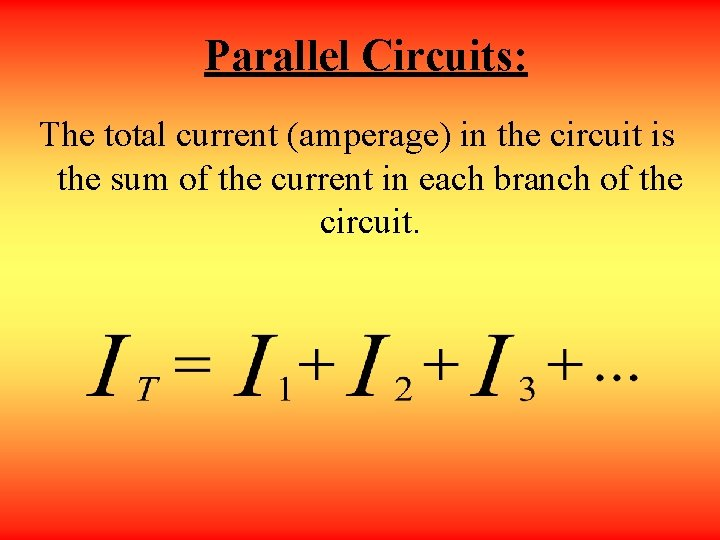Parallel Circuits: The total current (amperage) in the circuit is the sum of the current in each branch of the circuit.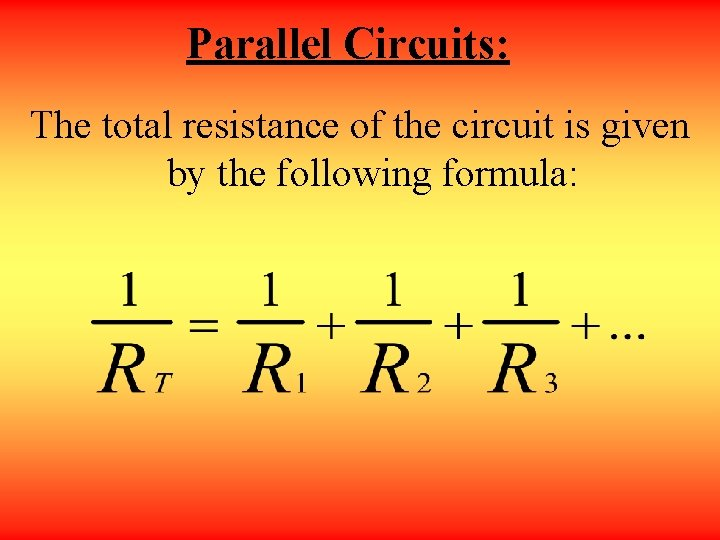Parallel Circuits: The total resistance of the circuit is given by the following formula:Parallel Circuits: The formula for total resistance can also be written:Parallel Circuits: The total voltage across the circuit is equal to the voltage across each resistor. VT = V 1 = V 2 = V 3 = …Two Birds on a Wire When the switch is closed, what happens to the birds? a) Bird 1 gets a shock and bird 2 does not b) Bird 2 gets a shock and bird 1 does not c) They both get a shock d) Neither gets a shock 12 V bulb switch ANSWER: Bird #1 will get shocked because there is a potential difference, whereas #2 will not.